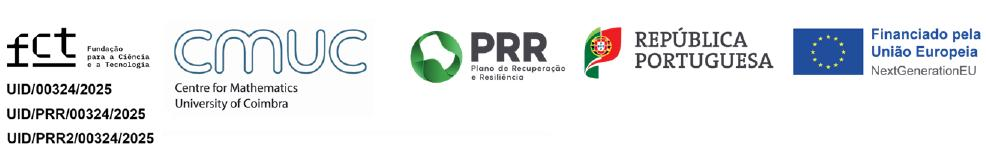Higher-order quantitative equational theories

Description:

Modern universal algebra frequently involves notions of equality that go beyond  the standard binary setting, in which equality either holds or does not hold.
In this talk we explore the idea of equality taking values from a quantale V, which covers the cases of (ultra)metric, fuzzy, and classical (in)equations  among others.

We start by presenting a V-equational system for linear lambda-calculus,  together with a proof that it is the internal language of a certain class of autonomous categories enriched over 'generalised metric spaces'. This is  analogous to the acclaimed result that simply typed lambda-calculus is the internal language of Cartesian closed categories.

We then drop the (often too strict) linearity constraint by adding graded modal  types which allow multiple uses of a resource in a controlled manner. We show  how to extend the previous V-equational system to a graded, sound and complete  one by using as interpretation what we call a Lipschitz exponential comonad.
We show additionally how to build such comonads canonically via a universal construction.

Finally we illustrate the applicability of our results with the derivation of metric equational systems (and corresponding models) for timed, quantum, and probabilistic computation.

References:
 Fredrik Dahlqvist and Renato Neves. An internal language for categories enriched over generalised metric spaces. In 30th EACSL Annual Conference on Computer Science Logic, 2022.
 Fredrik Dahlqvist and Renato Neves. The syntactic side of autonomous categories enriched over generalised metric spaces. LMCS (in print). arXiv preprint: arXiv.2208.14356.
 Fredrik Dahlqvist and Renato Neves. A Complete V-Equational System for Graded lambda-Calculus. arXiv preprint: arXiv.2304.02082.

Date:  2023-05-30
Start Time:   15:00
Speaker:  Renato Neves (Univ. do Minho)